The Google Sheets COUNTIFS function is used to count items in ranges with multiple test criteria.

It works like a COUNTIF function, but lets you include more than one conditional test.

Here’s an example with a small real estate example dataset: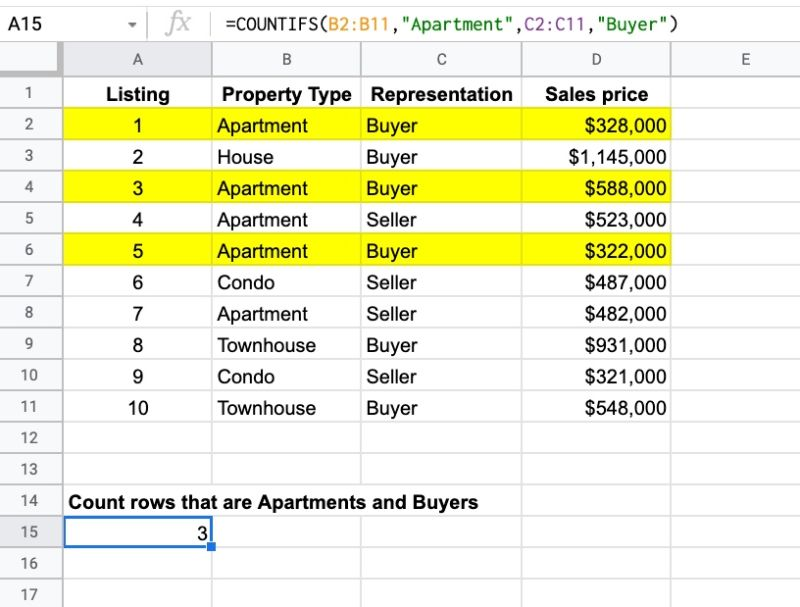How can we count the number of deals for apartments and buyers?

To count them, we use a COUNTIFS function:

`=COUNTIFS(B2:B11,"Apartment",C2:C11,"Buyer")`

There are three rows that are apartments AND buyers, highlighted in yellow.

## COUNTIFS Function Syntax

`=COUNTIFS(criteria_range1, criterion1, [criteria_range2, criterion2, ...])`

The COUNTIFS function takes a minimum of two arguments but can take more.

The arguments must always come in pairs:

• a range to count over, and
• a conditional test

The range is the data that you want to count across.

The criterion is the test you want to apply.

The ranges must all be the same dimension. In this example, you can clearly see that the orange and purple ranges are different sizes so the formula won’t work: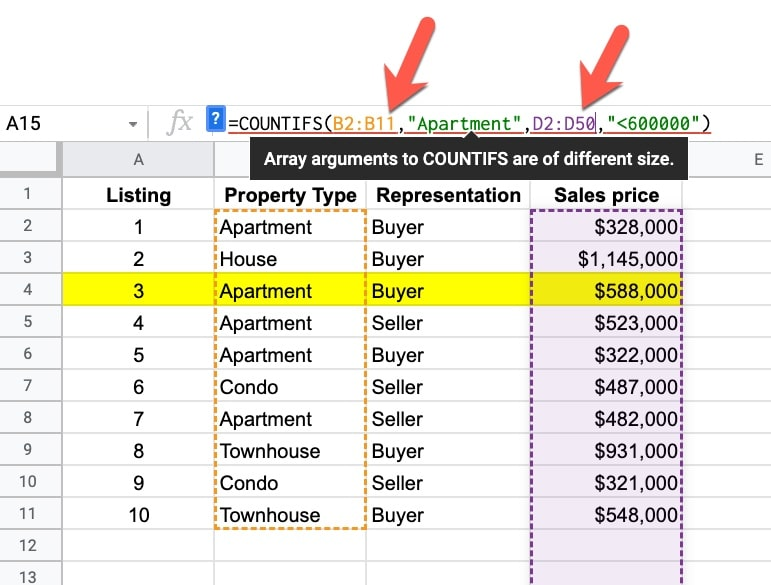Your formula will show a #VALUE! error:

“Array arguments to COUNTIFS are of different size.”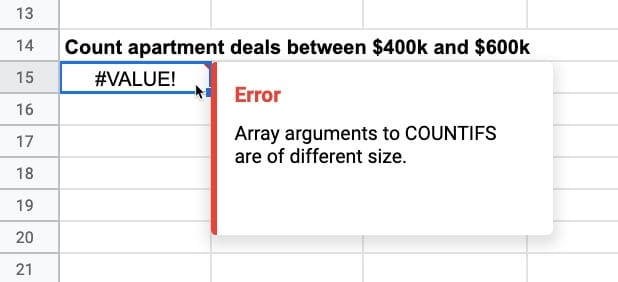Like its younger brother, the COUNTIF function, it’s part of the Math family of functions in Google Sheets.

## COUNTIFS Function Examples

### Text Criterion

Text criterion must be enclosed in double-quotes.

This formula counts rows with Apartments AND Buyers:

`=COUNTIFS(B2:B11,"Apartment",C2:C11,"Buyer")`### Numeric Criterion

For numeric tests looking for exact matches, the numbers are entered directly into COUNTIFS.

This formula will count rows with North AND value 100 (assumes a different dataset):

`=COUNTIFS(B2:B11,"North",C2:C11,100)`

### Logical Test Criterion

Now consider numeric criteria that involve logical tests. For these, you must use double-quotes.

For example, here’s the COUNTIFS to count Sellers AND values over \$400,000:

`=COUNTIFS(C2:C11,"Seller",D2:D11,">400000")`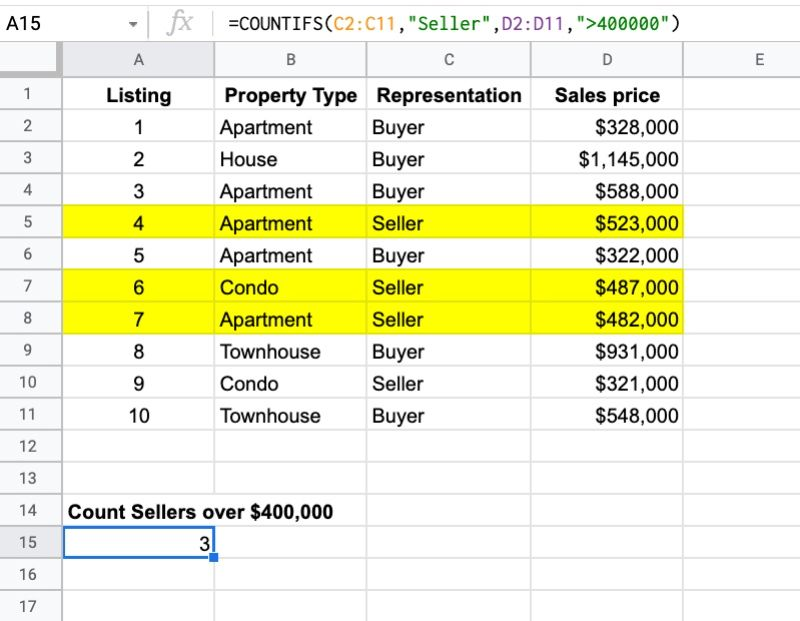The following logical operators can be used in COUNTIFS to create conditional tests:

• > greater than
• >= greater than or equal to
• < less than
• <= less than or equal to
• = equal to
• <> not equal to

They must be enclosed with double-quotes.

### Blank and Non-Blank Counts

Blank cells can be counted by setting the test criterion to `""`, e.g.

`=COUNTIFS(B2:B11,"Apartment",C2:C11,"")`

And non-blank cells can be counted by setting the test criterion to `"<>"`, e.g.

`=COUNTIFS(B2:B11,"Apartment",C2:C11,"<>")`

### Criterion In Same Column

Testing two criteria is possible but both criteria need to be true at the same time to count.

Considering a numeric example, we can use this COUNTIFS formula to find all deals between \$400k AND \$600k:

`=COUNTIFS(D2:D11,">400000",D2:D11,"<600000")`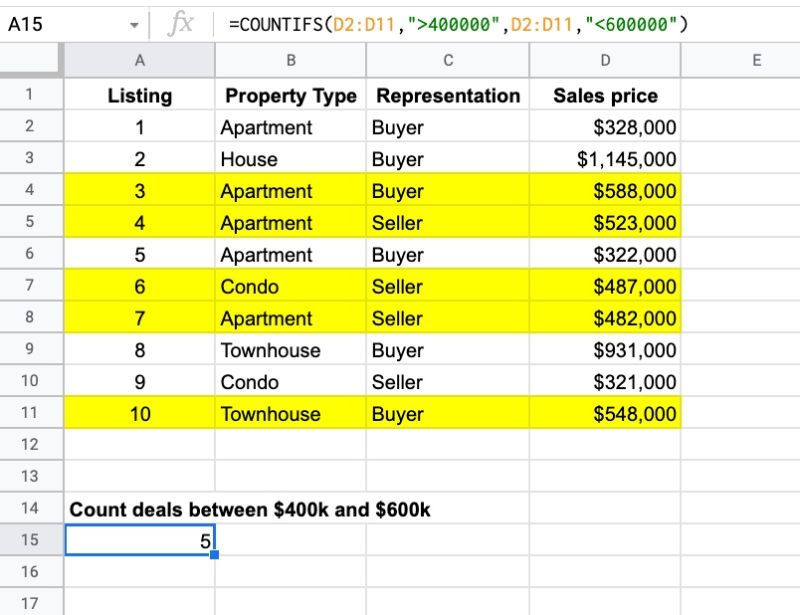It works because values can be greater than \$400k and less than \$600k at the same time, e.g. \$500k.

However, if you try something like this example:

`=COUNTIFS(B2:B11,"Apartment",B2:B11,"Condo")`

then you’ll get 0 as the result. A row can’t be classified as an Apartment AND a Condo at the same time.

In this case, we want to count when a row is either an Apartment OR a Condo.

We use two COUNTIF functions to do this:

`=COUNTIF(B2:B11,"Apartment") + COUNTIF(B2:B11,"Condo")`

### Three Criterion Example

To count Apartments between \$400k AND \$600k, we use this formula:

`=COUNTIFS(D2:D11 , ">400000" , D2:D11 , "<600000" , B2:B11 , "Apartment")`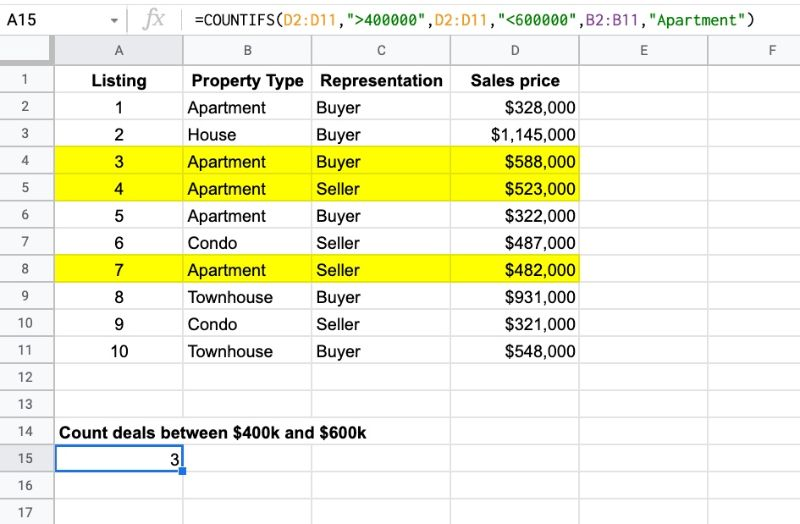Since it’s an AND condition, all three conditions must be true in order for it to be counted.